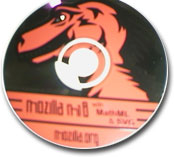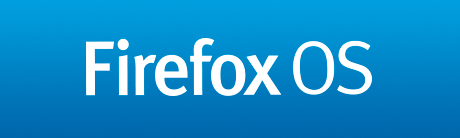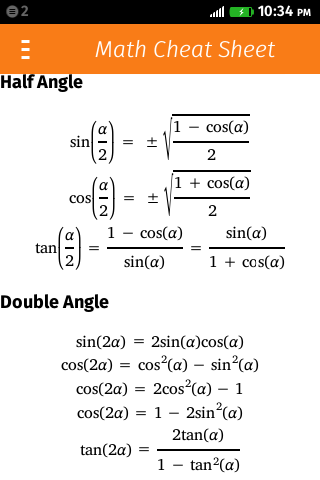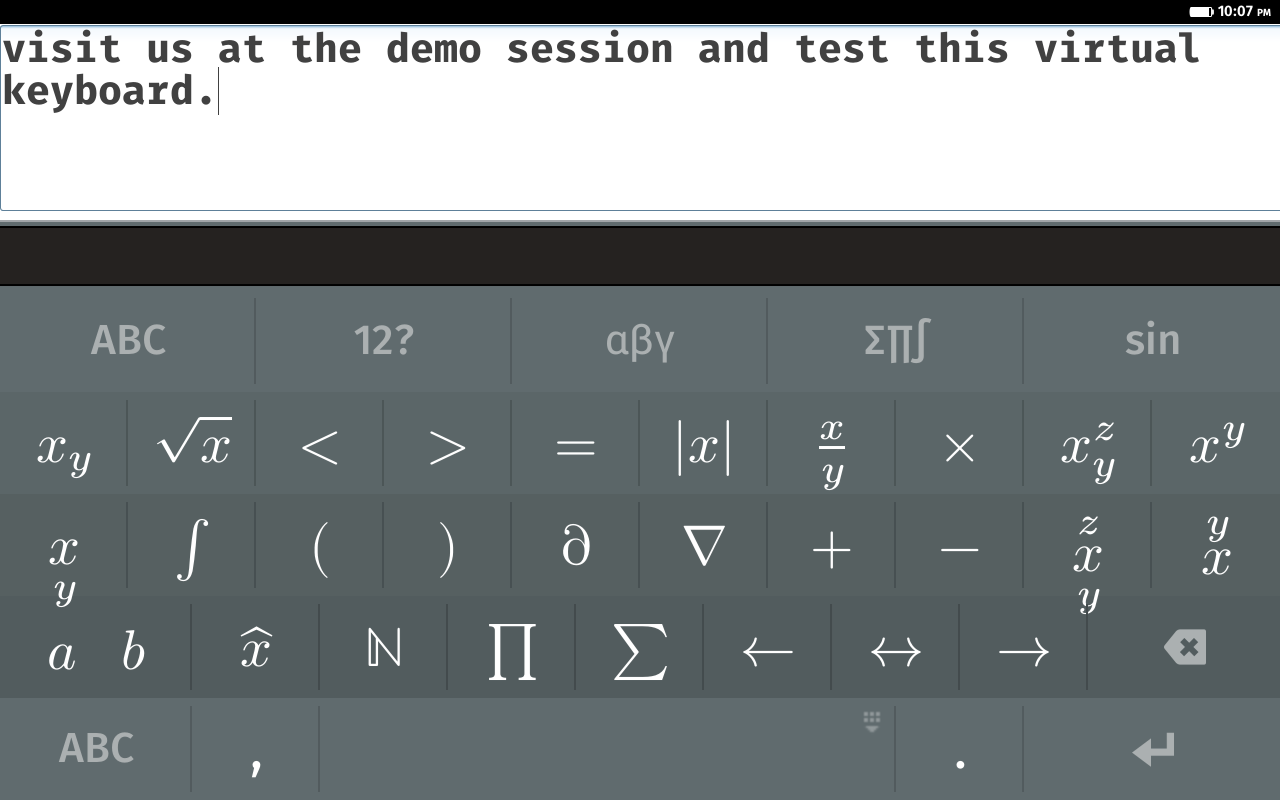# Firefox OS Web Apps for Science

## Firefox OS Web Apps for Science## Mozilla MathML Project

• Raniere Silva & Frédéric Wang
• ~10 volunteers
• 15 years anniversary
• Math formulae and moreMozilla CD at the 1st MathML Conference (October 2000)

## Firefox OS

• Mobile devices
• Open
• Web technologiesSmartphones, tablets and more.
Based on Free Software and Web standards.
Technologies familiar to Web developers
Gecko as the rendering engine and so MathML support.
Photo by Yortw

• HTML
• CSS
• SVG
• MathML
• Javascript
• Text Layout

## OpenType MATH table

• Microsoft Word, Modern TeX Engines, Gecko, WebKit
• Cambria Math, Latin Modern Math, STIX Math, WOFF

## TeXZilla

• Yet another LaTeX-to-MathML converter
• Internationalization
• Minimality
• Multiple Interfaces
• Integration in Mozilla Products
س = \frac{-ب \pm \sqrt{ب^٢ - ٤اج}}{٢ا}

$س = \frac\left\{-ب\pm\sqrt\left\{ب^٢-٤اج\right\}\right\}\left\{٢ا\right\}$

## Web Components


<x-tex display="block">
\Gamma(t) =
\frac{1}{t} \prod_{n=1}^\infty
\frac{\left(1+\frac{1}{n}\right)^t}{1+\frac{t}{n}} =
\frac{e^{-\gamma t}}{t} \prod_{n=1}^\infty
\left(1 + \frac{t}{n}\right)^{-1} e^{\frac{t}{n}}
</x-tex>

$\Gamma\left(t\right) = \lim_\left\{n \to \infty\right\} \frac\left\{n! \; n^t\right\}\left\{t \; \left(t+1\right)\cdots\left(t+n\right)\right\}= \frac\left\{1\right\}\left\{t\right\} \prod_\left\{n=1\right\}^\infty \frac\left\{\left\left(1+\frac\left\{1\right\}\left\{n\right\}\right\right)^t\right\}\left\{1+\frac\left\{t\right\}\left\{n\right\}\right\} = \frac\left\{e^\left\{-\gamma t\right\}\right\}\left\{t\right\} \prod_\left\{n=1\right\}^\infty \left\left(1 + \frac\left\{t\right\}\left\{n\right\}\right\right)^\left\{-1\right\} e^\left\{\frac\left\{t\right\}\left\{n\right\}\right\}$

## Firefox OS Apps

PackagedHosted
• App.zip
• index.html
• manifest.webapp
• css
• js
• img
• http://app.com
• index.html
• manifest.webapp
• css
• js
• img

## Firefox OS Manifest

        
{
"version": "1.0.0",
"name": "App",
"description": "App short description",
"launch_path": "/index.html",
"icons": {
"128": "/img/icon-128.png"
},
"developer": {
"name": "Your name or organization",
"url": "http://your-homepage-here.org"
},
"default_locale": "en"
}## Example of Firefox OS App (phone)## Example of Firefox OS App (tablet)## Example of Firefox OS App on other platforms## Math Suite## Thanks